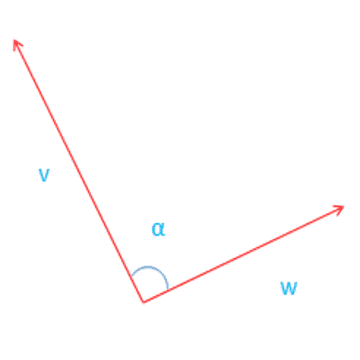# Two Dimensional Vector Angle Calculation

Using this Online Calculator, we can Calculate the Angle for two vectors v and w

 Vector v: , Vector w: .

 Angle: Degrees

In 2D (Two Dimensional) vector there is only one degree of freedom for 2D rotations.

If v and w are normalized

so that |v|=|w|=1, then

angle = inverse of cosine (v X w)

where: |v|,|w| is magnitude of v,w.In the case of two dimension vectors there is only one degree of freedom for rotations, and we can use the dot product to find the angle between 2 vectors.

If v and w are normalized so that |v|=|w| = 1

Therefore the angle θ between 2 vectors v and w can be find as

angle = arccos(|v||w|v•w)

where:

• = dot- product

arc cos = inverse of cosine function

|v|= magnitude of vector v

Mostly acos returns a value between 0 and π which is 0° and 180°.